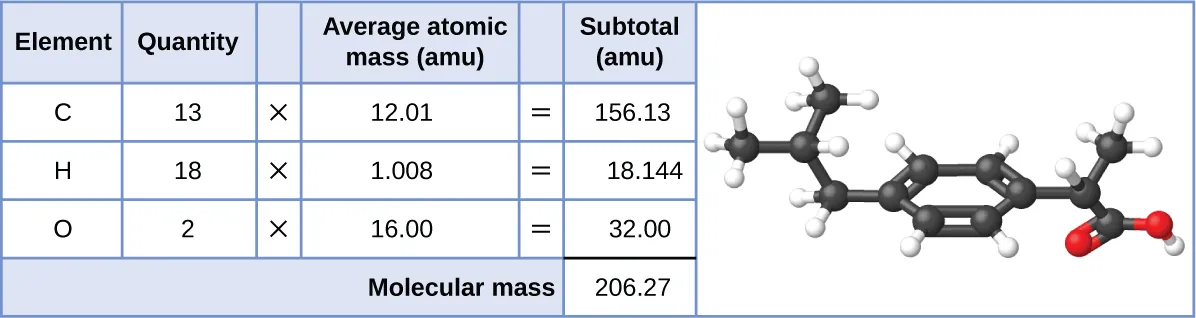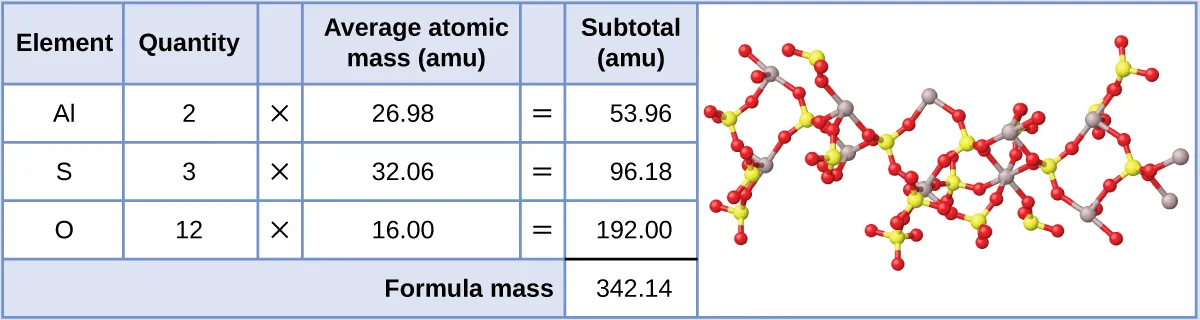Chemistry: Atoms First 2e

# 6.1Formula Mass

### Learning Objectives

By the end of this section, you will be able to:

• Calculate formula masses for covalent and ionic compounds

Many argue that modern chemical science began when scientists started exploring the quantitative as well as the qualitative aspects of chemistry. For example, Dalton’s atomic theory was an attempt to explain the results of measurements that allowed him to calculate the relative masses of elements combined in various compounds. Understanding the relationship between the masses of atoms and the chemical formulas of compounds allows us to quantitatively describe the composition of substances.

### Formula Mass

An earlier chapter of this text described the development of the atomic mass unit, the concept of average atomic masses, and the use of chemical formulas to represent the elemental makeup of substances. These ideas can be extended to calculate the formula mass of a substance by summing the average atomic masses of all the atoms represented in the substance’s formula.

#### Formula Mass for Covalent Substances

For covalent substances, the formula represents the numbers and types of atoms composing a single molecule of the substance; therefore, the formula mass may be correctly referred to as a molecular mass. Consider chloroform (CHCl3), a covalent compound once used as a surgical anesthetic and now primarily used in the production of the “anti-stick” polymer, Teflon. The molecular formula of chloroform indicates that a single molecule contains one carbon atom, one hydrogen atom, and three chlorine atoms. The average molecular mass of a chloroform molecule is therefore equal to the sum of the average atomic masses of these atoms. Figure 6.2 outlines the calculations used to derive the molecular mass of chloroform, which is 119.37 amu.

Figure 6.2 The average mass of a chloroform molecule, CHCl3, is 119.37 amu, which is the sum of the average atomic masses of each of its constituent atoms. The model shows the molecular structure of chloroform.

Likewise, the molecular mass of an aspirin molecule, C9H8O4, is the sum of the atomic masses of nine carbon atoms, eight hydrogen atoms, and four oxygen atoms, which amounts to 180.15 amu (Figure 6.3).

Figure 6.3 The average mass of an aspirin molecule is 180.15 amu. The model shows the molecular structure of aspirin, C9H8O4.

### Example 6.1

#### Computing Molecular Mass for a Covalent Compound

Ibuprofen, C13H18O2, is a covalent compound and the active ingredient in several popular nonprescription pain medications, such as Advil and Motrin. What is the molecular mass (amu) for this compound?

#### Solution

Molecules of this compound are comprised of 13 carbon atoms, 18 hydrogen atoms, and 2 oxygen atoms. Following the approach described above, the average molecular mass for this compound is therefore:Acetaminophen, C8H9NO2, is a covalent compound and the active ingredient in several popular nonprescription pain medications, such as Tylenol. What is the molecular mass (amu) for this compound?

151.16 amu

#### Formula Mass for Ionic Compounds

Ionic compounds are composed of discrete cations and anions combined in ratios to yield electrically neutral bulk matter. The formula mass for an ionic compound is calculated in the same way as the formula mass for covalent compounds: by summing the average atomic masses of all the atoms in the compound’s formula. Keep in mind, however, that the formula for an ionic compound does not represent the composition of a discrete molecule, so it may not correctly be referred to as the “molecular mass.”

As an example, consider sodium chloride, NaCl, the chemical name for common table salt. Sodium chloride is an ionic compound composed of sodium cations, Na+, and chloride anions, Cl, combined in a 1:1 ratio. The formula mass for this compound is computed as 58.44 amu (see Figure 6.4).

Figure 6.4 Table salt, NaCl, contains an array of sodium and chloride ions combined in a 1:1 ratio. Its formula mass is 58.44 amu.

Note that the average masses of neutral sodium and chlorine atoms were used in this computation, rather than the masses for sodium cations and chlorine anions. This approach is perfectly acceptable when computing the formula mass of an ionic compound. Even though a sodium cation has a slightly smaller mass than a sodium atom (since it is missing an electron), this difference will be offset by the fact that a chloride anion is slightly more massive than a chloride atom (due to the extra electron). Moreover, the mass of an electron is negligibly small with respect to the mass of a typical atom. Even when calculating the mass of an isolated ion, the missing or additional electrons can generally be ignored, since their contribution to the overall mass is negligible, reflected only in the nonsignificant digits that will be lost when the computed mass is properly rounded. The few exceptions to this guideline are very light ions derived from elements with precisely known atomic masses.

### Example 6.2

#### Computing Formula Mass for an Ionic Compound

Aluminum sulfate, Al2(SO4)3, is an ionic compound that is used in the manufacture of paper and in various water purification processes. What is the formula mass (amu) of this compound?

#### Solution

The formula for this compound indicates it contains Al3+ and SO42− ions combined in a 2:3 ratio. For purposes of computing a formula mass, it is helpful to rewrite the formula in the simpler format, Al2S3O12. Following the approach outlined above, the formula mass for this compound is calculated as follows:Calcium phosphate, Ca3(PO4)2, is an ionic compound and a common anti-caking agent added to food products. What is the formula mass (amu) of calcium phosphate?

310.18 amu

Order a print copy

As an Amazon Associate we earn from qualifying purchases.